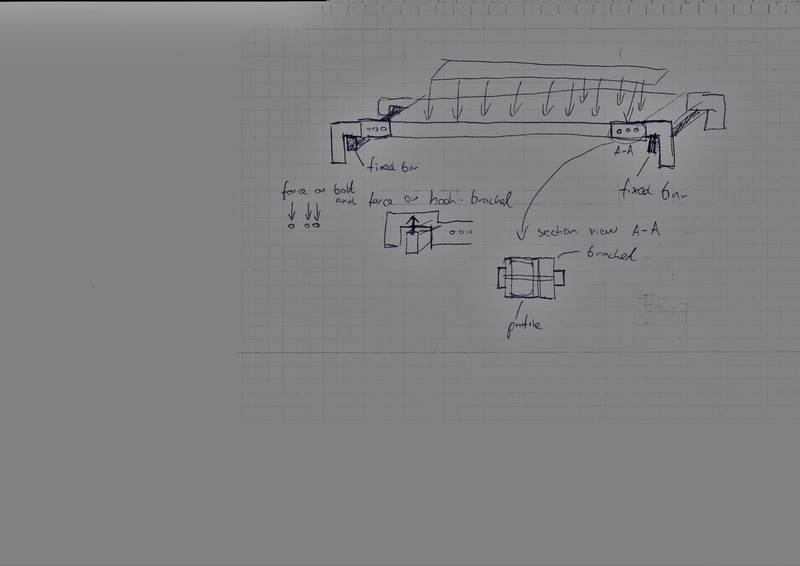# Forces in symmetrical construction

## Homework Statement

We have a slab with uniform distrubuted load on it W. All dimensions.
[/B]
I'm looking for the force on the bolts and force on the hook contact surface.## The Attempt at a Solution

My way to do it is to reduce the problem for 2D. But the problem is that i'm not sure how to solve this then. Because in 2D drawing we will be left with 6 bolts (3 each side) and 2 contact surface from hook).
The question I have:

1. The force that I will get is the half of the possible for this construction? Because I have other half of this construcion not calculated.

2. Is it not to many unknows? There is 6 force acting on each bolt and 2 pressure force acting on the bracket hook. How to find this forces? I know only dimensions and the pressure on the slab (the weigh of it is neglected)

Last edited by a moderator:

haruspex
Homework Helper
Gold Member
2020 Award
Whenever there are more constraints than are necessary to provide rigidity, it is not possible to determine all the forces precisely. You will have to make assumptions like things being perfectly symmetric, which will not be exactly the case.
For the four brackets resting on the bars, you might as well assume the load is distributed uniformly. In practice, it depends how the stiffness compares with the imperfections in the shapes. If the structures are extremely stiff and a pair of diametrically opposite brackets is slightly lower than the other pair, they will take most of the weight.

For the bolts, one bolt at a bracket would not constrain it, two would. The third gets us into the same problem as above. To proceed, you have to assume some degree of perfection. In this case, however, it would not be appropriate to assume uniform loading over the three. There are moments to consider. What equations can you develop for those?

The problem i Getting here is that i'm not sure if I can do things like that :

1 . Find C.O.G. for UDL. Attached there Point Force. (if the UDL working on Width= 300 mm and Length 400 mm. I just multiply by 300 mm to get the distributed force on member that is on the middle with span of 400 mm length.)

2. I trying to take the equilibrium around Y- Axis. So there will be force acting on the bracket from the bar from left side end right. I shouldn't take a bolts as a supports. So the force acting on the bolts should be moment multiply by arm. The bolt with longer arm have biggest force and that the bolt that I should check. I don't know only how to get the specific force acting on this bolt. Because it always be more unknows in Moment equilibrium. It won't be if i will assume that the force from poin

haruspex
Homework Helper
Gold Member
2020 Award